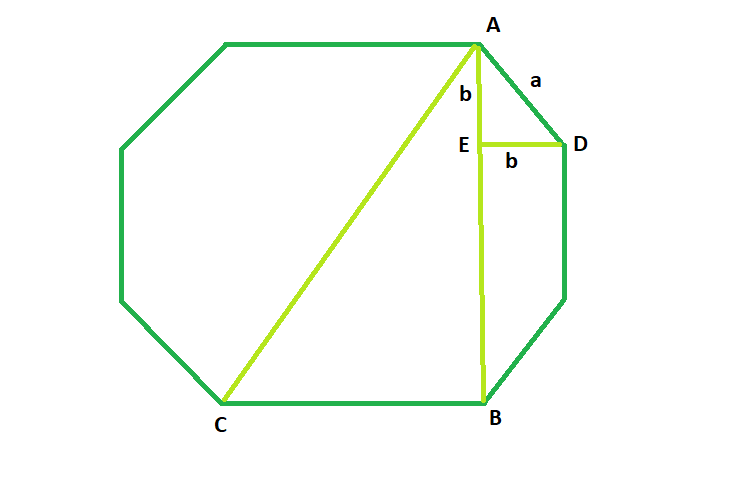# Length of the Diagonal of the Octagon

Given here is a regular octagon of side length a, the task is to find the length of it’s diagonal.

Examples:

```Input: a = 4
Output: 10.4525

Input: a = 5
Output: 13.0656

```

## Recommended: Please try your approach on {IDE} first, before moving on to the solution.Approach: From the diagram it is clear that,

AB^2 + BC^2 = AC^2
here, in triangle AED,
b^2 + b^2 = a^2

So, AB = a + 2b = a + √2a
and, BC = a
So, diagonal AC = a√(4 + 2√2)

Below is the implementation of the above approach:

## C++

 `// C++ Program to find the diagonal ` `// of the octagon ` `#include ` `using` `namespace` `std; ` ` `  `// Function to find the diagonal ` `// of the octagon ` `float` `octadiagonal(``float` `a) ` `{ ` ` `  `    ``// side cannot be negative ` `    ``if` `(a < 0) ` `        ``return` `-1; ` ` `  `    ``// diagonal of the octagon ` `    ``return` `a * ``sqrt``(4 + (2 * ``sqrt``(2))); ` `} ` ` `  `// Driver code ` `int` `main() ` `{ ` `    ``float` `a = 4; ` `    ``cout << octadiagonal(a) << endl; ` ` `  `    ``return` `0; ` `} `

## Java

 `// Java  Program to find the diagonal  ` `// of the octagon  ` `import` `java.util.*; ` `class` `solution ` `{ ` `   `  `// Function to find the diagonal  ` `// of the octagon  ` `static` `double` `octadiagonal(``double` `a)  ` `{  ` `   `  `    ``// side cannot be negative  ` `    ``if` `(a < ``0``)  ` `        ``return` `-``1``;  ` `   `  `    ``// diagonal of the octagon  ` `    ``return` `a * Math.sqrt(``4` `+ (``2` `* Math.sqrt(``2``)));  ` `}  ` `   `  `// Driver code  ` `public` `static` `void` `main(String args[]) ` `{  ` `    ``double` `a = ``4``;  ` `    ``System.out.println( octadiagonal(a));  ` `   `  `}  ` `} ` `//contributed by Arnab Kundu `

## Python3

 `# Python3 Program to find the diagonal ` `# of the octagon ` `import` `math ` `# Function to find the diagonal ` `# of the octagon ` `def` `octadiagonal(a): ` ` `  `    ``# side cannot be negative ` `    ``if` `(a < ``0``): ` `        ``return` `-``1``; ` ` `  `    ``# diagonal of the octagon ` `    ``return` `a ``*` `math.sqrt(``4` `+` `(``2` `*` `math.sqrt(``2``))) ` ` `  ` `  `# Driver code ` `if` `__name__``=``=``'__main__'``: ` `    ``a ``=` `4` `    ``print` `(octadiagonal(a)) ` ` `  `# This code is contributed by  ` `# Shivi_Aggarwal  `

## C#

 `// C# Program to find the diagonal  ` `// of the octagon  ` `using` `System; ` ` `  `class` `GFG ` `{ ` ` `  `// Function to find the diagonal  ` `// of the octagon  ` `static` `double` `octadiagonal(``double` `a)  ` `{  ` ` `  `    ``// side cannot be negative  ` `    ``if` `(a < 0)  ` `        ``return` `-1;  ` ` `  `    ``// diagonal of the octagon  ` `    ``return` `a * Math.Sqrt(4 +  ` `          ``(2 * Math.Sqrt(2)));  ` `}  ` ` `  `// Driver code  ` `public` `static` `void` `Main() ` `{  ` `    ``double` `a = 4;  ` `    ``Console.WriteLine(octadiagonal(a));  ` `}  ` `} ` ` `  `// This code is contributed  ` `// by inder_verma `

## PHP

 ` `

Output:

```10.4525
```

Attention reader! Don’t stop learning now. Get hold of all the important DSA concepts with the DSA Self Paced Course at a student-friendly price and become industry ready.

My Personal Notes arrow_drop_upProgram Analyst Trainee,Cognizant

If you like GeeksforGeeks and would like to contribute, you can also write an article using contribute.geeksforgeeks.org or mail your article to contribute@geeksforgeeks.org. See your article appearing on the GeeksforGeeks main page and help other Geeks.

Please Improve this article if you find anything incorrect by clicking on the "Improve Article" button below.